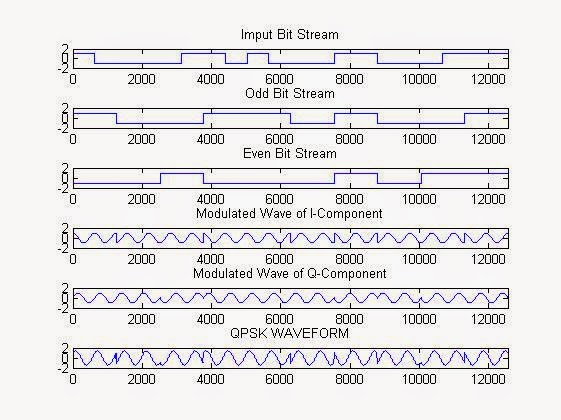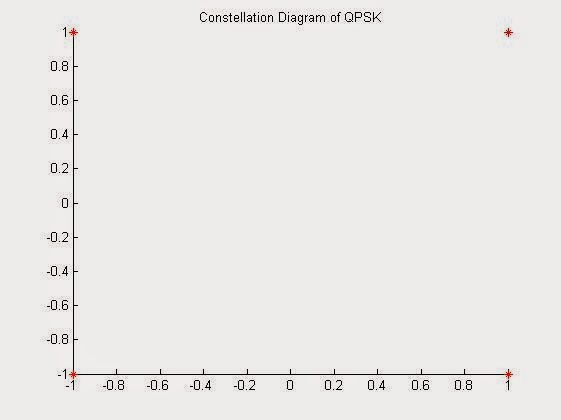# QPSK Digital Modulation Simulation Using MATLAB: Quadrature Phase Shift Keying

Quadrature Phase Shift Keying (QPSK) is one of  the type of phase shift keying. Unlike BPSK which send one bit information at an instance, QPSK is a modulation which sends two bits of digital information a time (without the use of another carrier frequency). They both are by the way DSBCS modulation scheme.

In a sense, QPSK is an expanded version from binary PSK where in a symbol consists of two bits and two orthonormal basis functions are used. A group of two bits is often called a 'dibit'. So, four dibits are possible. Each symbol carries same energy.

The above was just in introduction about what QPSK is, search over the internet to get more stuffs about it. You will get number of learning resources which explains you the A-Z of the theoretical aspect of it.

The process which we have followed while implementing on MATLAB is quite conventional.
First: get the bit stream.
Then Divide the bit stream in 2 bit streams: even & odd.
Then apply the BPSK techniques on these 2 bit streams separately.
Add the modulated waves to get the QPSK.

Another way to implement it in MATLAB or any other simulation software would be, to introduce a phase shift of  90 degree, on each pair of bit change. For more information just search Maxim Integrated QPSK tutorial, here you will get all the relevant equation information.

MATLAB Code For QPSK :

clc;
clear all;
close all;
cvx=input('Enter Length of Random Bit Sequence:');
d=round(rand(1,cvx))
l=cvx;
x=0:0.01:l*2*pi;
cc=cos(x);
cs=cos(x+pi/2);
k=length(cc);
k1=k/l;

for i=1:l
if(d(i)==0)
d(i)=-1;
i=i+1;
end
end

i=1;
j=1;
% To segrigate odd bit streams and even bit streams
while (i<l) && (j<l)
%half strem with double symbol duration
dd1(j)=d(i);
dd1(j+1)=d(i);
dd2(j)=d(i+1);
dd2(j+1)=d(i+1);
j=j+2;
i=i+2;
end
% to make bit streams cycle equivalent to sinusoidal waveformt=1;
for i=1:l
for j=1:k1
%k1 sample with 1 sine period
dd(t)=d(i);
d1(t)=dd1(i);
d2(t)=dd2(i);
t=t+1;
j=j+1;
end
i=i+1;
end

subplot(6,1,1);
stairs(dd);
axis([0 t -2 2]);
title('Imput Bit Stream');
subplot(6,1,2);
stairs(d1);
axis([0 t -2 2]);
title('Odd Bit Stream');
subplot(6,1,3);
stairs(d2);
axis([0 t -2 2]);
title('Even Bit Stream');

len=length(d1);
if(k<len)
len=k;
end

for i=1:len
qcc(i)=cc(i)*d1(i);
% odd streams multiplied with I waveform
qcs(i)=cs(i)*d2(i);
% even streams multiplied with Q waveform
i=i+1;
end

subplot(6,1,4);
plot(qcc);
axis([0 len -2 2]);
title('Modulated Wave of I-Component');
subplot(6,1,5);
plot(qcs);
axis([0 len -2 2]);
title('Modulated Wave of Q-Component');

qp=qcc+qcs;
subplot(6,1,6);
plot(qp);
axis([0 len -2 2]);
title('QPSK WAVEFORM');
figure, scatter(dd1,dd2,40,'*r');
title('Constellation Diagram of QPSK');

Results:QPSK MATLAB Simulation ResultQPSK Constellation Plot

1.1.2.